Want to share your content on R-bloggers? click here if you have a blog, or here if you don't.

By Chris Campbell – Senior Consultant, UK.

For many types of data, we have made a measurement of some variable that looks normally distributed. The middle value is the most likely, most values are similar to the middle value, and a few values may be larger or smaller.

```> uwt <- Theoph\$Wt[!duplicated(Theoph\$Subject)]
>
> # a histogram of weights
> hist(uwt)
>
> # calculate smoothed count density
> dwt <- density(uwt, bw = 4)
>
> # add density as a frequency
> lines(dwt\$x, dwt\$y * length(uwt) * 5, col = "red", lwd = 2)```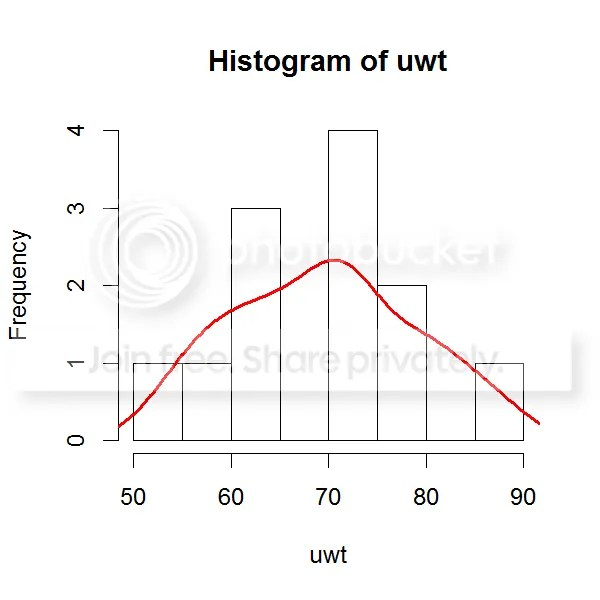For example, the mtcars data frame from the datasets package contains information about different types of cars. The fuel economy figures (mpg) look like they are normally distributed.

```> # a histogram of fuel economy
> hist(mtcars\$mpg)
>
> # calculate smoothed count density
> dmpg <- density((mtcars\$mpg))
>
> # add density as a frequency
> lines(dmpg\$x, dmpg\$y * nrow(mtcars) * 5, col = "red", lwd = 2)```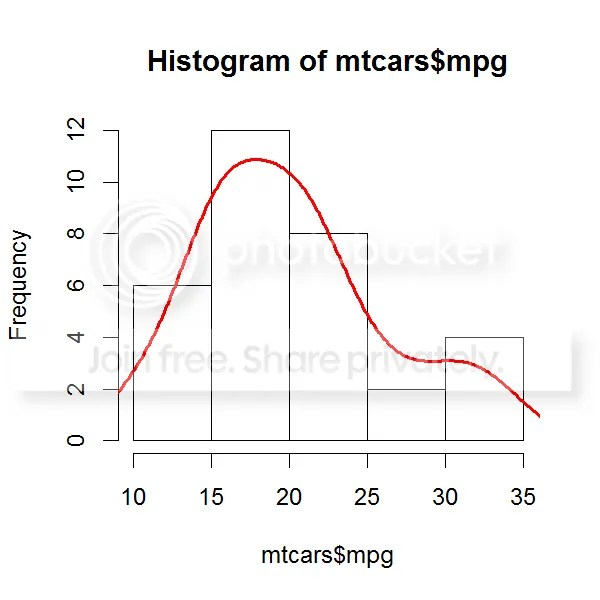If we wanted to predict a fuel economy figure for a similar type of car to those analysed, then we might expect the number to be around 20 give or take a few. But what if we wanted to guess whether it’d be automatic or manual?

```> # automatic or manual transmission
> unique(mtcars\$am)
 1 0
>
> # categorical data (two categories)
> barplot(table(mtcars\$am))```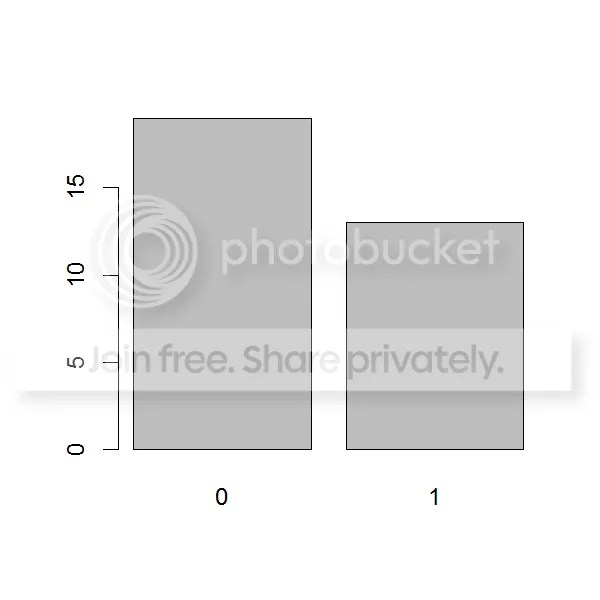There are only two possible values we’d want to predict; 0 for automatic, or 1 for manual. Predicting 0.5 or -1 wouldn’t be useful, as there are only two categories to choose from.

We can represent categorical data in R using factors. Factors are a type of numeric column where every possible category (or level) has a character label. By default, these levels are created alphabetically from the levels in the data object. We can update these using the labels argument. Factors can have any number of levels.

```> levels(factor(mtcars\$am))
 "0" "1"
>
> # the factor class signifies that this is categorical data
> mtcars\$am <- factor(mtcars\$am,
+     levels = c(0, 1),
+     labels = c("automatic", "manual"))
>
> # updated labels can be assigned to each level
> levels(mtcars\$am)
 "automatic" "manual"
>
> mtcars\$cyl <- factor(mtcars\$cyl)
> levels(mtcars\$cyl)
 "4" "6" "8"```

A linear model is a formalized way of examining relationships between variables. This rule of thumb can be used to make predictions about how the system will behave in the future. Linear models can include continuous and categorical independent variables. The function lm returns an object containing information about this model fit.

```> lm_mpg_disp_am <- lm(mpg ~ disp + am,
+    data = mtcars)
> lm_mpg_disp_am

Call:
lm(formula = mpg ~ disp + am, data = mtcars)

Coefficients:
(Intercept)         disp     ammanual
27.84808     -0.03685      1.83346

>
> # summary statistics of the model
> s_mda <- summary(lm_mpg_disp_am)
> s_mda

Call:
lm(formula = mpg ~ disp + am, data = mtcars)

Residuals:
Min      1Q  Median      3Q     Max
-4.6382 -2.4751 -0.5631  2.2333  6.8386

Coefficients:
Estimate Std. Error t value Pr(>|t|)
(Intercept) 27.848081   1.834071  15.184 2.45e-15 ***
disp        -0.036851   0.005782  -6.373 5.75e-07 ***
ammanual     1.833458   1.436100   1.277    0.212
---
Signif. codes:  0 ‘***’ 0.001 ‘**’ 0.01 ‘*’ 0.05 ‘.’ 0.1 ‘ ’ 1

Residual standard error: 3.218 on 29 degrees of freedom
Multiple R-squared:  0.7333,    Adjusted R-squared:  0.7149
F-statistic: 39.87 on 2 and 29 DF,  p-value: 4.749e-09```

By default we include an intercept in the model. The meaning of the intercept depends on how we structure the model, but in this case, an automatic car with a displacement of 0 is predicted to have a fuel economy of 27.8 mpg. This is also a nice example of why extrapolating to the boundaries of a model is not necessarily a good idea!

This model also suggests that heavier cars have a lower fuel economy (the gradient of disp is negative), and manual cars have a higher fuel economy (the manual category has a positive effect on the intercept). However, the standard error of ammanual is quite large compared to the Estimate, so we’re not sure about whether this coefficient is meaningful.

What about cars with a higher number of cylinders?

```> # the effect of the number of cylinders
> lm_mpg_disp_cyl <- lm(mpg ~ disp + cyl,
+    data = mtcars)
>
> # looks a little better
> summary(lm_mpg_disp_cyl)

Call:
lm(formula = mpg ~ disp + cyl, data = mtcars)

Residuals:
Min      1Q  Median      3Q     Max
-4.8304 -1.5873 -0.5851  0.9753  6.3069

Coefficients:
Estimate Std. Error t value Pr(>|t|)
(Intercept) 29.53477    1.42662  20.703  < 2e-16 ***
disp        -0.02731    0.01061  -2.574  0.01564 *
cyl6        -4.78585    1.64982  -2.901  0.00717 **
cyl8        -4.79209    2.88682  -1.660  0.10808
---
Signif. codes:  0 ‘***’ 0.001 ‘**’ 0.01 ‘*’ 0.05 ‘.’ 0.1 ‘ ’ 1

Residual standard error: 2.95 on 28 degrees of freedom
Multiple R-squared:  0.7837,    Adjusted R-squared:  0.7605
F-statistic: 33.81 on 3 and 28 DF,  p-value: 1.906e-09```

Cars with more cylinders had a lower fuel economy (mpg). However, the model cannot distinguish between cars with 6 and 8 cylinders. We could consider dropping a level from cyl so that the categories are ‘4’ and ‘>4′.

We can visualize relationships with continuous variates quite clearly with a scatter plot. We might prefer boxplots to compare categories. Factors work nicely for determining how points should be plotted. Elements of a vector can be selected using the index of that vector.

```> # an aside on colouring plots
> # six digits with a hash signifies an RGB hex code (from 00 to FF)
> # the 7th and 8th digits are transparency (aka alpha)
> cols <- c("#EE334466", "#2255EE66")
>
> # we can select elements of a vector using square brackets...
> cols
 "#EE334466"
> cols
 "#2255EE66"
>
> # ... as many times as we want
> cols[c(1, 1, 2, 2)]
 "#EE334466" "#EE334466" "#2255EE66" "#2255EE66"
>
> # factors can be coerced to numeric
> as.numeric(mtcars\$am)
 2 2 2 1 1 1 1 1 1 1 1 1 1 1 1 1 1 2 2 2 1 1 1 1 1 2 2 2 2 2 2 2
> cols[mtcars\$am]
 "#2255EE66" "#2255EE66" "#2255EE66" "#EE334466" "#EE334466" "#EE334466"
 "#EE334466" "#EE334466" "#EE334466" "#EE334466" "#EE334466" "#EE334466"
 "#EE334466" "#EE334466" "#EE334466" "#EE334466" "#EE334466" "#2255EE66"
 "#2255EE66" "#2255EE66" "#EE334466" "#EE334466" "#EE334466" "#EE334466"
 "#EE334466" "#2255EE66" "#2255EE66" "#2255EE66" "#2255EE66" "#2255EE66"
 "#2255EE66" "#2255EE66"```

We can use this property to reliably colour each point in a plot using one colour for each level. We can use the predict function to make predictions at a range of positions in the model variates.

```> plot(mpg ~ disp, data = mtcars,
+     col = cols[mtcars\$am],
+     pch = 16)
>
> # we can use models to predict values for new data
> # for example a sequence of points
> d1 <- seq(50, 500, length = 10)
> p1 <- predict(lm_mpg_disp_am,
+     newdata = list(disp = rep(d1, 2),
+         am = rep(levels(mtcars\$am), each = 10)))
>
> # matlines can be used to add a matrix of lines to a plot
> # this shows us the shape of the first model
> # linear models (often) produce straight lines
> matlines(d1, matrix(p1, ncol = 2, byrow = FALSE),
+     col = cols, lty = 1, lwd = 2)```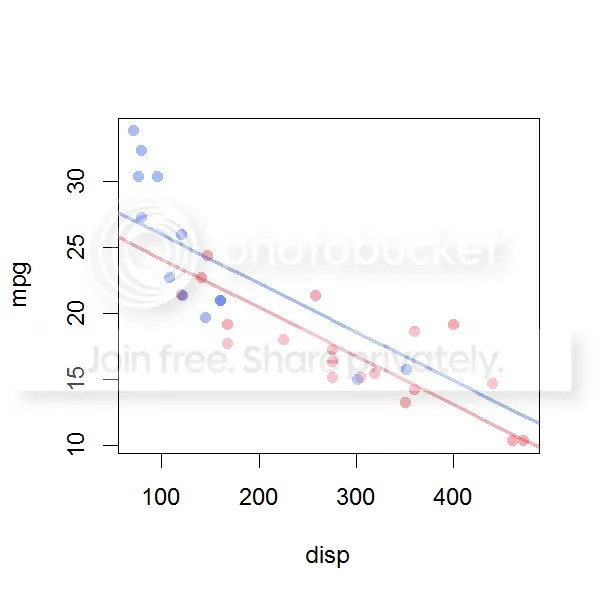However, data are not always normally distributed! For example, our data may follow some other distribution, or be limited in the possible range of values expected (probabilities must fall between 0 and 1) or even the type of value expected (counts are always integers, categories are not numbers)!

```> toluene <- read.csv("toluene.csv")
> hist(toluene\$Toluene_ppm)
>
> # random count data
> rpois(5, lambda = 3)
 5 2 1 0 3
>
> require(faraway)
> # probabilities
> plot(I(damage/6) ~ temp, data = orings)```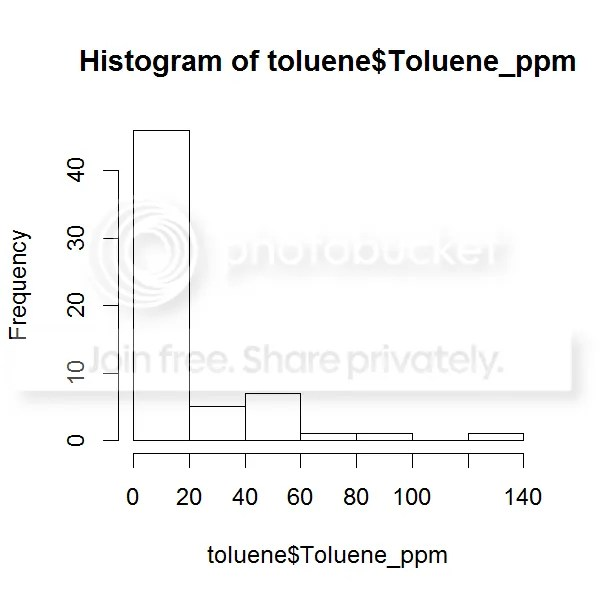Generalised linear models are very similar to linear models. We almost use the same syntax with the function glm, and in addition, we provide a family. The family describes the error structure of the data.

```> glm_mpg_disp_am <- glm(mpg ~ disp + am,
+    data = mtcars, family = gaussian)
>
> summary(glm_mpg_disp_am)

Call:
glm(formula = mpg ~ disp + am, family = gaussian, data = mtcars)

Deviance Residuals:
Min       1Q   Median       3Q      Max
-4.6382  -2.4751  -0.5631   2.2333   6.8386

Coefficients:
Estimate Std. Error t value Pr(>|t|)
(Intercept) 27.848081   1.834071  15.184 2.45e-15 ***
disp        -0.036851   0.005782  -6.373 5.75e-07 ***
ammanual     1.833458   1.436100   1.277    0.212
---
Signif. codes:  0 ‘***’ 0.001 ‘**’ 0.01 ‘*’ 0.05 ‘.’ 0.1 ‘ ’ 1

(Dispersion parameter for gaussian family taken to be 10.35453)

Null deviance: 1126.05  on 31  degrees of freedom
Residual deviance:  300.28  on 29  degrees of freedom
AIC: 170.46

Number of Fisher Scoring iterations: 2

> summary(lm_mpg_disp_am)

Call:
lm(formula = mpg ~ disp + am, data = mtcars)

Residuals:
Min      1Q  Median      3Q     Max
-4.6382 -2.4751 -0.5631  2.2333  6.8386

Coefficients:
Estimate Std. Error t value Pr(>|t|)
(Intercept) 27.848081   1.834071  15.184 2.45e-15 ***
disp        -0.036851   0.005782  -6.373 5.75e-07 ***
ammanual     1.833458   1.436100   1.277    0.212
---
Signif. codes:  0 ‘***’ 0.001 ‘**’ 0.01 ‘*’ 0.05 ‘.’ 0.1 ‘ ’ 1

Residual standard error: 3.218 on 29 degrees of freedom
Multiple R-squared:  0.7333,    Adjusted R-squared:  0.7149
F-statistic: 39.87 on 2 and 29 DF,  p-value: 4.749e-09

>
> class(lm_mpg_disp_am)
 "lm"
> class(glm_mpg_disp_am)
 "glm" "lm"```

In fact, with the gaussian family, they are the same. A glm is simply a linear model working with transformed data. The data is transformed first by a link function. For the gaussian family, the link function is the identity transformation (f(x) = x). For other families, the link function is an appropriate transformation, such that fitting a linear model is meaningful. For probability data, the link function is the logit transformation (f(x) = ln(x/(1-x))).

The orings dataset consists of the number of damaged o-rings on launches of the Challenger space shuttle. On each launch there were six rings. Following each launch the rings were inspected.

```> head(orings)
temp damage
1   53      5
2   57      1
3   58      1
4   63      1
5   66      0
6   67      0
> table(orings\$damage)

0  1  5
16  6  1```

We can create a glm of events (oring failures) from a number of trials for a given temperature.

```> glm_damg_temp <- glm(cbind(damage, 6 - damage) ~ temp,
+     data = orings, family = "binomial")
>
> # each record contains the result of 6 trials
> summary(glm_damg_temp)

Call:
glm(formula = cbind(damage, 6 - damage) ~ temp, family = "binomial",
data = orings)

Deviance Residuals:
Min       1Q   Median       3Q      Max
-0.9529  -0.7345  -0.4393  -0.2079   1.9565

Coefficients:
Estimate Std. Error z value Pr(>|z|)
(Intercept) 11.66299    3.29626   3.538 0.000403 ***
temp        -0.21623    0.05318  -4.066 4.78e-05 ***
---
Signif. codes:  0 ‘***’ 0.001 ‘**’ 0.01 ‘*’ 0.05 ‘.’ 0.1 ‘ ’ 1

(Dispersion parameter for binomial family taken to be 1)

Null deviance: 38.898  on 22  degrees of freedom
Residual deviance: 16.912  on 21  degrees of freedom
AIC: 33.675

Number of Fisher Scoring iterations: 6```

The output is very similar to that of lm. Large estimates and small standard errors give us confidence that the parameters are meaningful. If we want to know the the probability of an event, we can use the predict function. We need to specify that we are interested in a response, rather than a prediction of the transformed variable.

```> t1 <- seq(40, 85, length = 50)
> pp1 <- predict(glm_damg_temp, newdata = list(temp = t1),
+     type = "response")
1         2         3         4         5         6
0.9531867 0.9434843 0.9319147 0.9181819 0.9019707 0.8829568
>
> # we can visualize the effect of the continuous variable temperature
> plot(I(damage/6) ~ temp, data = orings, xlim = c(40, 90))
> lines(t1, pp1, col = "red", lwd = 2)```If we want to extrapolate the probability of 6 events at a new temperature, such as the day of the launch, 31’F we can multiply the probability of one event (assuming the events are independent).

```> ppp <- predict(glm_damg_temp, newdata = list(temp = 31),
+     type = "response")
> ppp^6
1
0.958926```

If this model is valid, it predicts a 96% chance of 6/6 oring failures at 31’F.

By changing the family, dependent data which is not normally distributed can be investigated with less bias, and producing more meaningful predictions than by linear modelling. For more help with R, stats and modelling, contact [email protected].